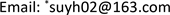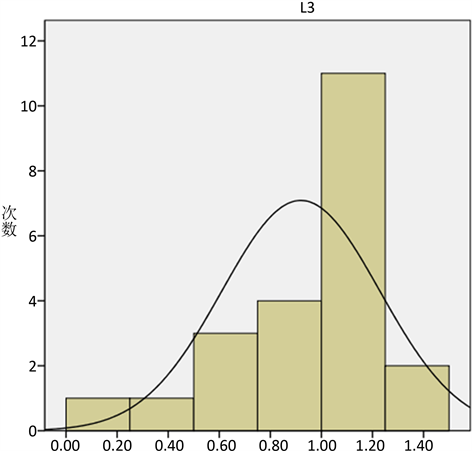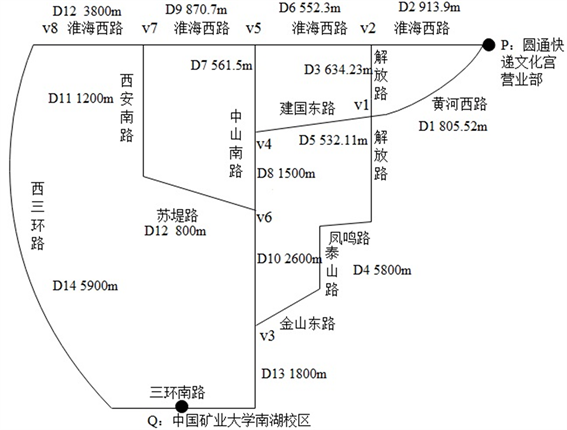﻿ 不确定因素下一类物流车最优路径模型的建立与求解 Solution and Establishing of a Selection of Optimal Route for Logistics Vehicles Models under the Uncertain Environment

Vol.06 No.07(2017), Article ID:22545,10 pages
10.12677/AAM.2017.67104

Solution and Establishing of a Selection of Optimal Route for Logistics Vehicles Models under the Uncertain Environment

Ruonan Shen, Youhui Su*

College of Mathematics and Physics, Xuzhou Institute of Technology, Xuzhou JiangsuReceived: Oct. 9th, 2017; accepted: Oct. 23rd, 2017; published: Oct. 31st, 2017ABSTRACT

This paper has established the optimal path model under uncertain factors as the subject of research, i.e., from Culture Palace Business Department of Xuzhou Yuantong Express to Nanhu Campus of China University of Mining and Technology. By using the additive property of the normal distribution, the expression of the normal distribution for travel time of each path has been obtained, which is standardized to derive the expression of travel time. When the probability of reaching the end point is determined, the optimal path is the path with the shortest travel time. On this basis, the optimal path algorithm based on depth-first search is designed, and seven different paths are obtained by MATLAB programming. The minimum travel time and the optimal path are obtained.

Keywords:Optimal Path, Normal Distribution Additive, Convolution Formula, Depth-First Search Algorithm1. 引言

2. 不确定性条件下的最优路径

2.1. 模型假设

1) 假设行驶时间是在满足通达率的情况下、一定范围的随机变量；

2) 假设在交通网络节点上的时间不存在；

3) 各个路段的行驶时间变量相互独立；

4) 假设各个车道之间的行驶状况的影响不存在。

2.2. 模型的准备

2.3. 不确定因素下最优路径模型的建立

${p}_{{T}_{k}}\left({t}_{k}\right)=\frac{1}{\sqrt{2\text{π}}{\sigma }_{k}}×\mathrm{exp}\left\{-\frac{{\left({t}_{k}-{\mu }_{k}\right)}^{2}}{2{\sigma }_{k}^{2}}\right\}。$

${T}_{\left(2\right)}={T}_{1}+{T}_{2}\sim N\left({\mu }_{1}+{\mu }_{2},{\sigma }_{1}^{2}+{\sigma }_{2}^{2}\right),$Figure 1. When the traffic is smooth, the travel time distribution curve of the road D3

${T}_{\left(n\right)}={T}_{1}+{T}_{2}+\cdots +{T}_{n},$

${T}_{\left(n\right)}\sim N\left({\mu }_{1}+{\mu }_{2}+\cdots +{\mu }_{n},{\sigma }_{1}^{2}+{\sigma }_{2}^{2}+\cdots +{\sigma }_{n}^{2}\right),$

${p}_{T}\left(t\right)=\frac{1}{\sqrt{2\pi \left({\sigma }_{1}^{2}+{\sigma }_{2}^{2}+\cdots +{\sigma }_{n}^{2}\right)}}×\mathrm{exp}\left\{-\frac{{\left(t-\sum _{i=1}^{n}{\mu }_{i}\right)}^{2}}{2\left({\sigma }_{1}^{2}+{\sigma }_{2}^{2}+\cdots +{\sigma }_{n}^{2}\right)}\right\}.$

${T}_{i}\sim N\left(\sum _{j=1}^{k}{\mu }_{ij},\sum _{j=1}^{k}{\sigma }_{ij}^{2}\right),$

$\frac{{t}_{i}-\sum _{j=1}^{k}{\mu }_{ij}}{\sqrt{\sum _{j=1}^{k}{\sigma }_{ij}^{2}}}\sim N\left(0,1\right).$

$\Phi \left(\frac{{t}_{i}-\sum _{j=1}^{k}{\mu }_{ij}}{\sqrt{\sum _{j=1}^{k}{\sigma }_{ij}^{2}}}\right)=p,$

${t}_{i}=\sum _{j=1}^{k}{\mu }_{ij}+{u}_{p}×\sqrt{\sum _{j=1}^{k}{\sigma }_{ij}^{2}},$

$\mathrm{min}\text{\hspace{0.17em}}\text{\hspace{0.17em}}\sum _{j=1}^{k}{\mu }_{ij}+{u}_{p}×\sqrt{\sum _{j=1}^{k}{\sigma }_{ij}^{2}}.$

3. 基于深度优先搜索算法的最优路径求解

3.1. 基于深度优先搜索的最优路径算法

3.1.1. 深度优先搜索算法思想  

1) 访问搜索到的顶点 ${v}_{0}$

2) 将 ${v}_{0}$ 顶点的visited数组中的元素值置为1；

3) 搜索 ${v}_{0}$ 点所有未被访问的邻接点，若该邻接点存在，则从此邻接点开始递归，即开始相同的访问和搜索；若不存在，则返回到上一顶点。

3.1.2. 最优路径算法的理论综述

Step1：

Step2：

Step3：

Step4：

$\Phi \left(\frac{{t}_{i}-\sum _{j=1}^{k}{\mu }_{ij}}{\sqrt{\sum _{j=1}^{k}{\sigma }_{ij}^{2}}}\right)=p\text{\hspace{0.17em}}.$

Step5：

3.2. 算法的实际应用

3.3. 最优路径算法的求解Figure 2. Traffic Simplified Chart

$P\to {v}_{2}\to {v}_{1}\to {v}_{4}\to {v}_{6}\to {v}_{3}\to Q\text{\hspace{0.17em}}.$

4. 结论

Solution and Establishing of a Selection of Optimal Route for Logistics Vehicles Models under the Uncertain Environment[J]. 应用数学进展, 2017, 06(07): 861-870. http://dx.doi.org/10.12677/AAM.2017.67104

1. 1. 张梦颖. 不确定因素下路径规划问题研究[D]: [博士学位论文]. 合肥: 中国科学技术大学, 2016: 21-31.

2. 2. 王君. 不确定因素下车辆路径问题建模及优化方法研究[D]: [博士学位论文]. 天津: 天津大学, 2013: 2-8.

3. 3. 于福才. 物流企业配送中心选址研究——以绥化申通快递为例[J]. 企业导报, 2014(24): 20-21.

4. 4. 雷超. 不确定条件下的移动设施规划与调度优化研究[D]: [博士学位论文]. 北京: 清华大学, 2015: 1-10.

5. 5. 廖伟. 考虑共同配送和能耗的车辆路径问题优化研究[D]: [博士学位论文]. 成都: 西南交通大学, 2014: 2-8.

6. 6. 刘佳倩, 朱家明. 不确定条件下交通网络的动态最优路径求解算法[J]. 上海工程技术大学学报, 2016, 30(3): 246-251.

7. 7. 陆海良, 郁钢, 单宇翔. 基于改进遗传算法的车辆路径优化算法[J]. 工业控制计算机, 2015, 28(10): 74-75.

8. 8. 许克平, 曾明月. 彭圆红基于不确定因素下的Floyd算法改进[J]. 中国科技信息, 2016(18): 49-50.

9. 9. 杨阳. 基于深度优先搜索的混成系统有界可达性分析[D]: [硕士学位论文]. 南京: 南京大学, 2013: 4-14.

10. 10. 唐青松. 深度优先算法在创建树形结构中的应用研究[J]. 计算机技术与发展, 2014(9): 226-229.Figure 1. Traffic Simplified Chart

function possiablePaths = findPath(Graph, partialPath, destination, partialWeight)

% findPath按深度优先搜索所有可能的从partialPath出发到destination的路径，这些路径中不包含环路

% Graph: 路网图，非无穷或0表示两节点之间直接连通，矩阵值就为路网权值

% partialPath: 出发的路径，如果partialPath就一个数，表示这个就是起始点

% destination: 目标节点

% partialWeight: partialPath的权值，当partialPath为一个数时，partialWeight为0

pathLength = length(partialPath);

lastNode = partialPath(pathLength); %得到最后一个节点

nextNodes = find(0

GLength = length(Graph);

possiablePaths = [];

if lastNode == destination

% 如果lastNode与目标节点相等，则说明partialPath就是从其出发到目标节点的路径，结果只有这一个，直接返回

possiablePaths = partialPath;

possiablePaths(GLength + 1) = partialWeight;

return;

elseif length( find( partialPath == destination ) ) ~= 0

return;

end

%nextNodes中的数一定大于0,所以为了让nextNodes(i)去掉，先将其赋值为0A=zeros(10,10);

for i=1:length(nextNodes)

if destination == nextNodes(i)

%输出路径

tmpPath = cat(2, partialPath, destination); %串接成一条完整的路径

tmpPath(GLength + 1) = partialWeight + Graph(lastNode, destination); %延长数组长度至GLength+1, 最后一个元素用于存放该路径的总路阻

possiablePaths( length(possiablePaths) + 1 , : ) = tmpPath;

nextNodes(i) = 0;

elseif length( find( partialPath == nextNodes(i) ) ) ~= 0

nextNodes(i) = 0;

end

end

nextNodes = nextNodes(nextNodes ~= 0); %将nextNodes中为0的值去掉，因为下一个节点可能已经遍历过或者它就是目标节点

for i=1:length(nextNodes)

tmpPath = cat(2, partialPath, nextNodes(i));

tmpPsbPaths = findPath(Graph, tmpPath, destination, partialWeight + Graph(lastNode, nextNodes(i)));

possiablePaths = cat(1, possiablePaths, tmpPsbPaths);

end

clear all;

close all;

clc;

%输入均值

A=inf(10,10);

A(1,2)=7;A(2,1)=7;A(1,3)=3;A(3,1)=3;A(2,3)=3;A(3,2)=3;A(2,4)=3;A(4,2)=3;A(2,6)=22;

A(6,2)=22;A(3,5)=3;A(5,3)=3;A(4,5)=3;A(5,4)=3;A(4,7)=8;A(7,4)=8;A(5,8)=3;A(8,5)=3;

A(6,7)=9;A(7,6)=9;A(6,10)=4;A(10,6)=4;A(7,8)=10;A(8,7)=10;A(8,9)=9;A(9,8)=9;A(10,9)=16;

A(9,10)=16;

for i=1:10

A(i,i)=0;

end

TotalMean=findPath(A, 1, 10, 0)%每条路径总均值

%输入方差

B=inf(10,10);

B(1,2)=0.0643;B(2,1)=0.0643;B(1,3)=0.0476;B(3,1)=0.0476;B(2,3)=0.0624;B(3,2)=0.0624;

B(2,4)=0.0519;B(4,2)=0.0519;B(2,6)=0.0939;B(6,2)=0.0939;B(3,5)=0.0639;B(5,3)=0.0639;

B(4,5)=0.0519;B(5,4)=0.0519;B(4,7)=0.06;B(7,4)=0.06;B(5,8)=0.0813;B(8,5)=0.0813;

B(6,7)=0.0735;B(7,6)=0.0735;B(6,10)=0.0779;B(10,6)=0.0779;B(7,8)=0.0779;B(8,7)=0.0779;

B(8,9)=0.0806;B(9,8)=0.0806;B(10,9)=0.092;B(9,10)=0.092;

for i=1:10

B(i,i)=0;

end

TotalVar=findPath(B, 1, 10, 0)%每条路径总方差

%计算行驶时间

T=TotalMean(:,11)+1.96*sqrt(TotalVar(:,11));

1. 打开知网页面http://kns.cnki.net/kns/brief/result.aspx?dbPrefix=WWJD

2. 打开知网首页http://cnki.net/